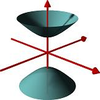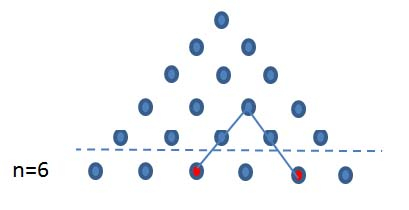#### You may also like### A Close Match

Can you massage the parameters of these curves to make them match as closely as possible?### Prime Counter

A short challenge concerning prime numbers.### The Right Volume

Can you rotate a curve to make a volume of 1?

# Combinations of Two

##### Age 16 to 18 ShortChallenge LevelCan you use this diagram to prove that the number of different pairs of objects which can be chosen from six objects, $^6C_2$, is $$1 + 2 + 3 + 4 + 5?$$
Generalise this to show that the number of ways of choosing pairs from $n$ objects is
$$^nC_2 = 1 + 2 + ...+ (n-1) = \frac{1}{2}n (n - 1).$$
Did you know ... ?
The sum of the first $n$ whole numbers is called a triangle number because this sum can be represented geometrically by a triangular array of dots. The sum is easily found by working out the number of dots in the parallelogram formed by putting two triangular arrays side by side.